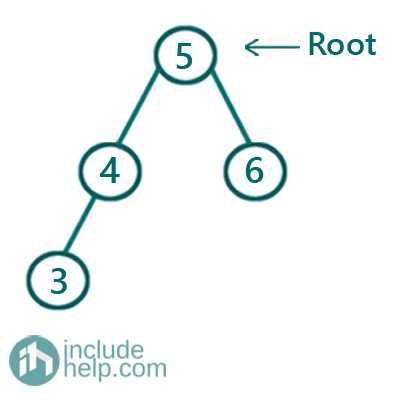# Insertion in a Binary Search Tree | Set 2

In this article, we are going to see how to insert a node into a given binary search tree?
Submitted by Radib Kar, on September 17, 2020

Example:

Say the given tree is: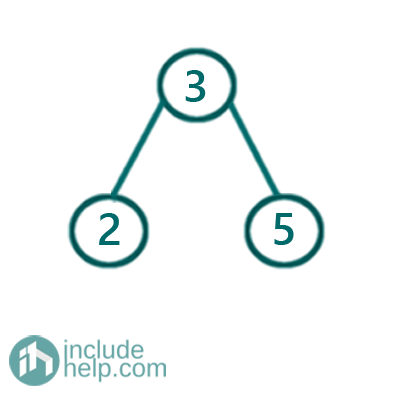After inserting value 4,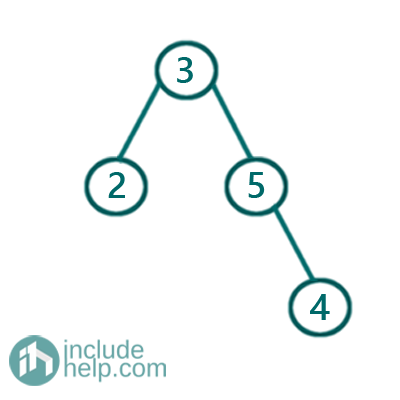Algorithm to insert a node in a given binary tree

1. We need to insert the node to a node with one child only. That's why we need to reach the correct node to insert. Now the question is to which one is the correct node where we can insert the node.
2. To find the correct Node we need search in the tree. So we keep checking with the current node value against the value to be inserted. If the value to be inserted is less than the current node then move to the left subtree, else move to the right subtree.

Below is the pictorial representation on how can we insert nodes to a given tree.

Say the given tree is,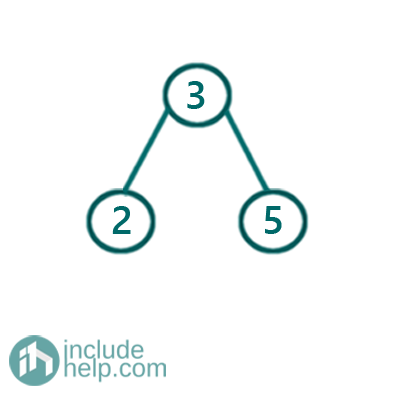Node to be inserted: 4

Iteration 1: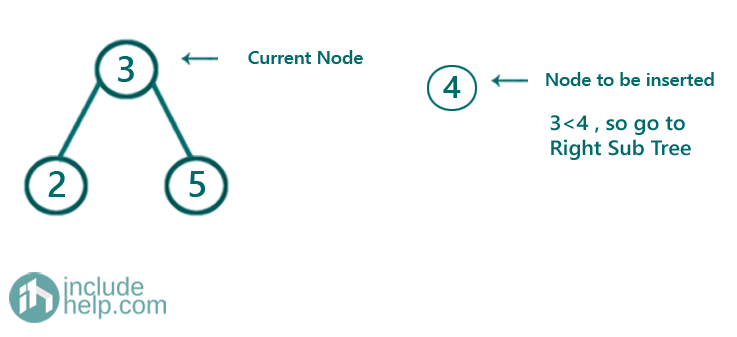Iteration 2: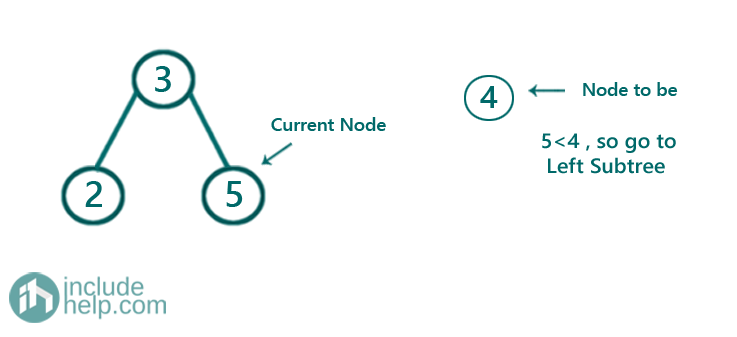Iteration 3: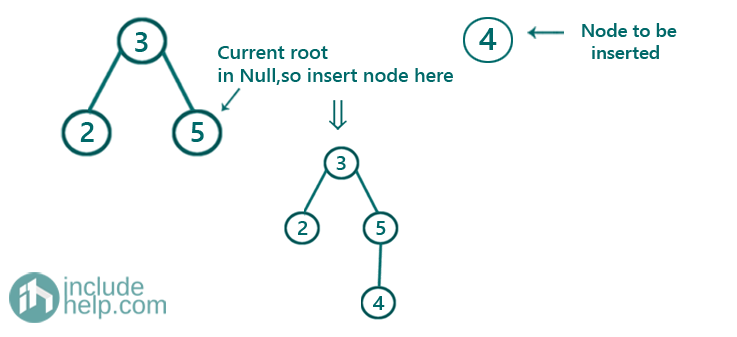Below is the C++ implementation.

```#include <bits/stdc++.h>
using namespace std;

// tree node is defined
class TreeNode {
public:
int val;
TreeNode* left;
TreeNode* right;
TreeNode(int data)
{
val = data;
left = NULL;
right = NULL;
}
};

TreeNode* insert(TreeNode* root, int Key)
{ //base case
// if root is NULL
if (!root)
return new TreeNode(Key); //this is the new root

//if root value is more than the Key,
//then we need to insert in the left subtree only
if (root->val > Key)
//recursively insert in the left subtree
root->left = insert(root->left, Key);
//if root value is less than the Key,
//then we need to insert in the right subtree only
else if (root->val < Key)
//recursively insert in the right subtree
root->right = insert(root->right, Key);

return root;
}

void inorder(TreeNode* root)
{
if (!root)
return;
inorder(root->left);
cout << root->val << " ";
inorder(root->right);
}

int main()
{
TreeNode* root = NULL;

cout << "Enter the first node\n";
int data;
cin >> data;

root = new TreeNode(data);

cout << "Root created...\n";
while (true) {
cout << "If you want to enter more nodes press y, else press n\n";
string str;
cin >> str;
if (str == "n" || str == "N")
break;
cout << "Enter node value\n";
cin >> data;
root = insert(root, data);
cout << "Node inserted...\n";
}
cout << "Insertion finished successfully\n";

cout << "Inorder traversal of the created tree is...\n";
inorder(root);

cout << "\n";

return 0;
}
```

Output:

```Enter the first node
5
Root created...
If you want to enter more nodes press y, else press n
y
Enter node value
4
Node inserted...
If you want to enter more nodes press y, else press n
y
Enter node value
3
Node inserted...
If you want to enter more nodes press y, else press n
y
Enter node value
6
Node inserted...
If you want to enter more nodes press y, else press n
n
Insertion finished successfully
Inorder traversal of the created tree is...
3 4 5 6
```

Dry Run:

Initially, Root is NULL

1) Insert 52) Insert 4

Since 4 is less than the root value thus it goes to the left subtree and since that's NULL. So we insert there.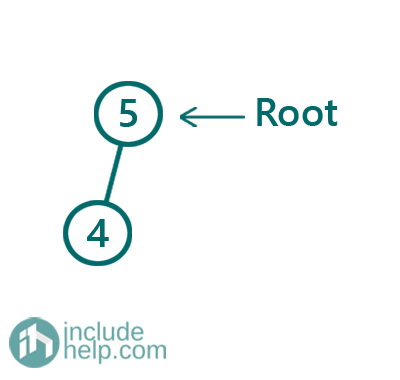4) Insert 3

Since 3 is less than the root value thus it goes to the left subtree and then it's smaller than 4(current root). Then again we go to the left subtree which is NULL, so we insert there.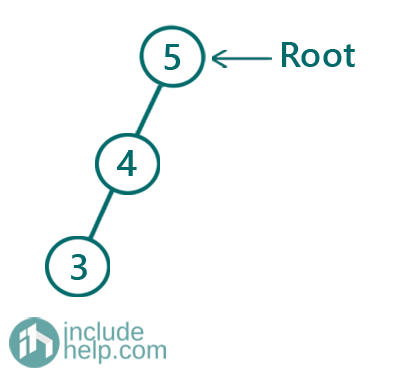5) Insert 6

The value is greater than the root value thus go to the right subtree which is NULL, thus we insert there.